#DAY 15
0
Modern Web

## 你是說...樹嗎?

https://openprocessing.org/sketch/90192
https://openprocessing.org/sketch/1009781

## 何謂遞迴

``````let people = 10; // 輸入10人
race(people);
function race(N){
// 進行一輪淘汰賽
N--;
if(N > 5) race(N);
else alert('共有' + N + '人入圍') // 輸出5人
}
``````

## 遞迴存在的意義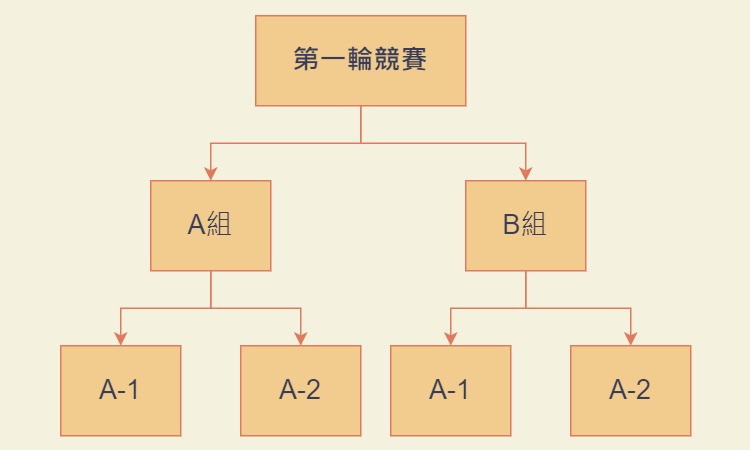## 樹狀圖

1. 樹枝本身
2. 有父節點（靠近根部）
3. 有子節點（靠近葉子）

``````let Tree = function(x, y, times){
// 樹枝本身(的屬性)
this.startX = x;
this.startY = y;
this.vectorX = 0;
this.vectorY = -10; // 每一節只有10像素，並且只會向正上方成長

// 根據輸入條件判斷是否創建新的樹枝
if(times > 0){
let endX = x + this.vectorX;
let endY = y + this.vectorY;
this.son = new Tree(x + vectorX, y + vectorY, times - 1);
}
else{
alert("too many isn't it?"); // 在樹枝的最末稍停止遞迴時，會觸發
}
};
let justAVar = new Tree(10, 10, 100);
``````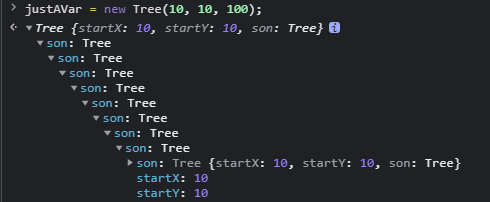``````let Tree = function(father, x, y, r, theta, times){
this.father = father;
this.startX = x;
this.startY = y;
this.r = r;
this.theta = theta;
if(times > 0){
let endX = x + r * Math.cos(theta / 180 * Math.PI);
let endY = y + r * Math.sin(theta / 180 * Math.PI);
this.son = [new Tree(this, endX, endY, r / 2, theta - 30, times - 1),
new Tree(this, endX, endY, r / 2, theta + 30, times - 1)];
}
else{
// 如果要讓末端開花結果，就寫在這
}

this.Draw = function(x = this.startX, y = this.startY,
r = this.r, theta = this.theta){
context.beginPath();
context.moveTo(x, y);
context.lineTo(x + r * Math.cos(theta / 180 * Math.PI),
y + r * Math.sin(theta / 180 * Math.PI));
context.lineWidth = 1 + r/50;
context.strokeStyle = 'rgba(179, 198, 213, 1)';
context.stroke();
// 如果有子樹枝，就繼續呼叫所有的子樹枝
if(this.son) this.son.forEach(branch => branch.Draw()); // 遞迴
};
};
``````

`myTree = new Tree(window, WIDTH/2, HEIGHT, HEIGHT/4, -90, 10);`

``````function Redraw(){
Clear(context);
myTree.draw();
}
``````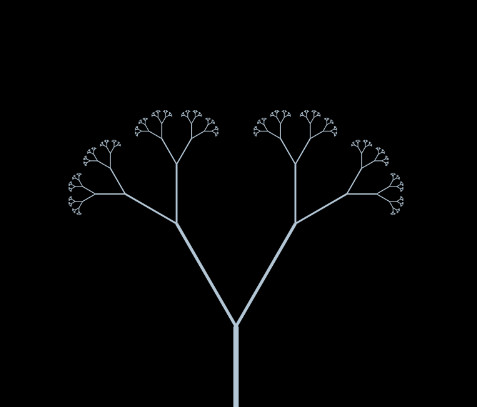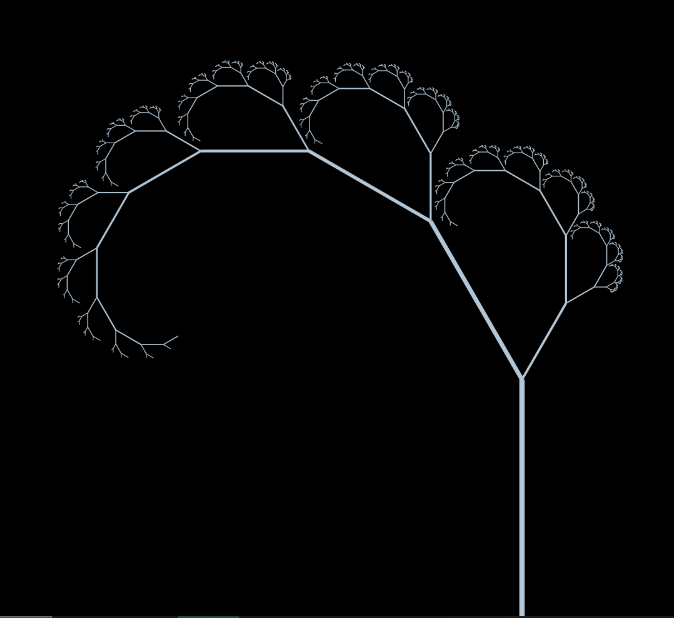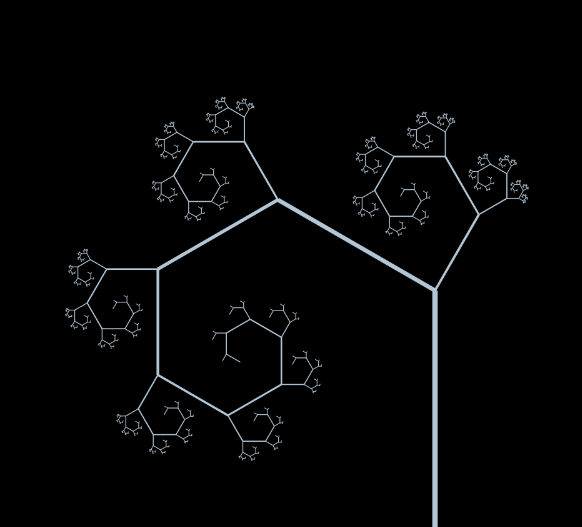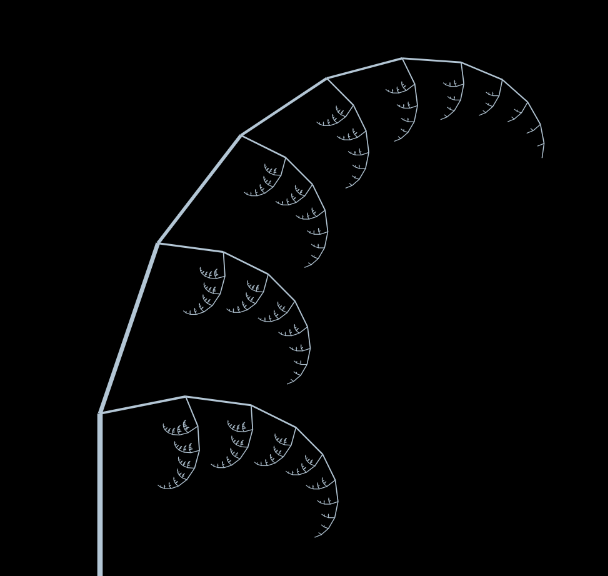demo 明天再補上吧！早點睡休息重要